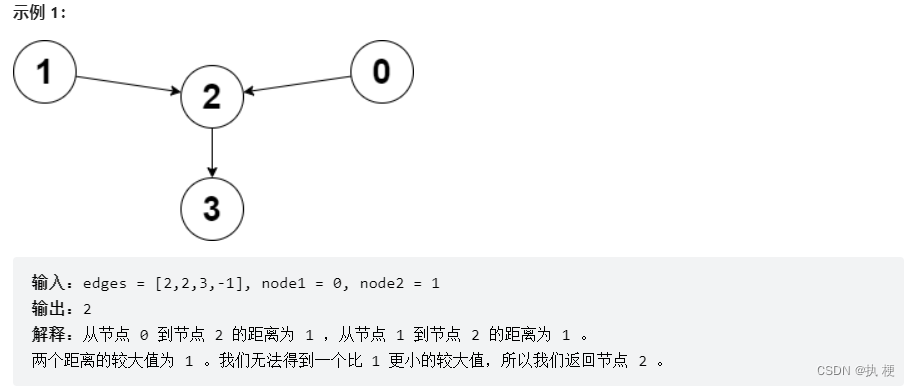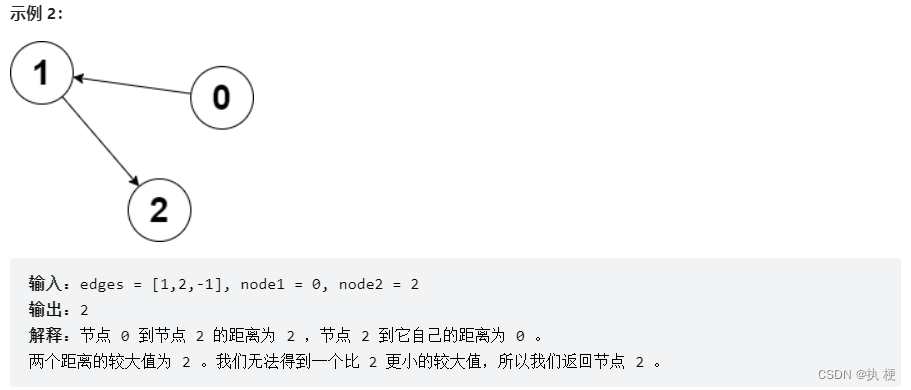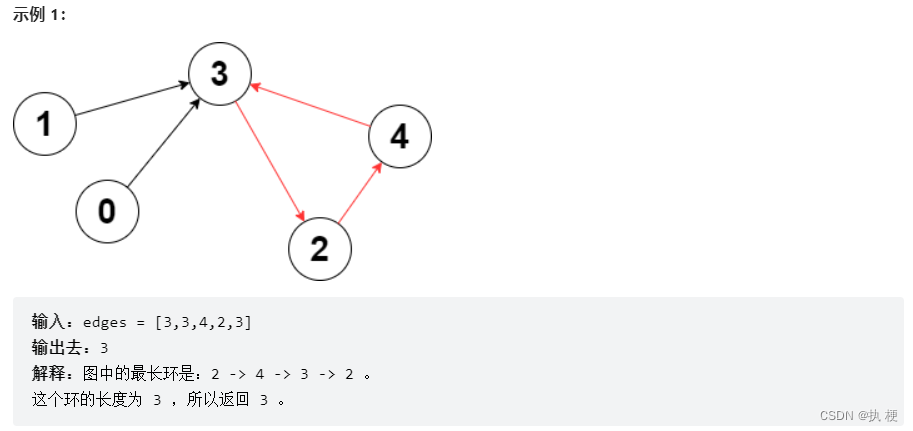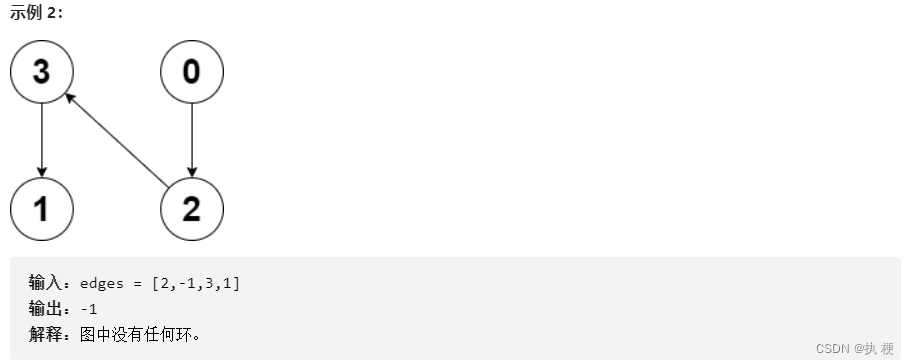> 文档中心 > 【周赛复盘】LeetCode第304场单周赛

# 【周赛复盘】LeetCode第304场单周赛

### 目录

• 1.使数组中所有元素都等于零
• 1）题目描述
• 2）原题链接
• 3）思路解析
• 4）模板代码
• 5）算法与时间复杂度
• 2、分组的最大数量
• 1）题目描述
• 2）原题链接
• 3）思路解析
• 4）模板代码
• 5）算法与时间复杂度
• 3.找到离给定两个节点最近的节点
• 1）题目描述
• 2）原题链接
• 3）思路解析
• 4）模板代码
• 5）算法与时间复杂度
• 4.图中最长环
• 1）题目描述
• 2）原题链接
• 3）思路解析
• 4）模板代码
• 5）算法与时间复杂度
• 5、周赛总结

# 1.使数组中所有元素都等于零

## 1）题目描述

• 选出一个正整数 xx 需要小于或等于 nums最小非零 元素。
• nums 中的每个正整数都减去 x

## 2）原题链接

LeetCode.6132. 使数组中所有元素都等于零

## 3）思路解析

• ( 1 ) (1) 很明显，贪心的思考我们应该每次选取数组中最小的非0元素作为x，然后将所有元素慢慢变为0。问题将变化为数组中存在多少个非0不同元素

## 4）模板代码

 public int minimumOperations(int[] nums) { Set<Integer> set=new HashSet<>(); int n=nums.length; for (int num : nums) {     if (num != 0) set.add(num); } return set.size();    }

## 5）算法与时间复杂度

算法：贪心
时间复杂度：遍历一次数组，复杂度为 O ( n ) O(n)

# 2、分组的最大数量

## 1）题目描述

• i 个分组中的学生总成绩 小于(i + 1) 个分组中的学生总成绩，对所有组均成立（除了最后一组）。
• i 个分组中的学生总数 小于(i + 1) 个分组中的学生总数，对所有组均成立（除了最后一组）。
返回可以形成的 最大 组数。

## 2）原题链接

LeetCode.6133. 分组的最大数量

## 3）思路解析

• ( 1 ) (1) 后面的组不仅要保证人比前面的多，还要保证总成绩高于前面前面的组。那么很明显，我们可以让成绩差的人去人少的组，成绩好的人去人多的组，这样就一定能保证符合条件。然后我们以组人数分别为1,2,3…这样分下去，看最多能分多少组。这里相当于是一个等差数列，我们可以二分 出答案。

## 4）模板代码

 public int maximumGroups(int[] arr) { int n=arr.length; int l=0,r=100000; while (l<r){     int mid=l+r+1>>1;     long v= (long) mid *(mid+1)/2;     if(v>n) r=mid-1;     else l=mid; } return r;    }

## 5）算法与时间复杂度

算法：贪心 脑筋急转弯 二分
时间复杂度：二分复杂度为 O ( l o g n ) O(logn)

# 3.找到离给定两个节点最近的节点

## 1）题目描述## 2）原题链接

LeetCode.6134. 找到离给定两个节点最近的节点

## 3）思路解析

• ( 1 ) (1) 首先很明显，答案节点必须是node1node2都能到达的节点，我们可以对两个结点分别做一次bfs，把遇到的节点分别放到set中。
• ( 2 ) (2) 使用dist数组统计到达每个点的距离，由于答案求的是较大值最小化，所以首先两个结点都能到达的结点的距离我们需要取max
• ( 3 ) (3) 最后对dist进行遍历，找到两个set都存储过的点，然后这些找到dist最小的结点。

## 4）模板代码

Map<Integer,Integer> map=new HashMap<>();    int N=100010;    int[] dist=new int[N];    int ans=Integer.MAX_VALUE;    Set<Integer> s1=new HashSet<>();    Set<Integer> s2=new HashSet<>();    int jie=-1;    public int closestMeetingNode(int[] edges, int node1, int node2) { int n=edges.length; for (int i = 0; i <n; i++) {     add(i,edges[i]); } bfs(node1,true); bfs(node2,false); for (int i = 0; i < n; i++) {     if (s1.contains(i)&&s2.contains(i)){  if (dist[i]<ans){      ans=dist[i];      jie=i;  }     } } return jie;    }    void bfs(int start,boolean f){ Queue<Integer> q=new LinkedList<>(); boolean[] st=new boolean[N]; st[start]=true; q.offer(start); int x=0; while (!q.isEmpty()){     int size=q.size();     while (size-->0){  int curr=q.poll();  if (f) s1.add(curr);  else s2.add(curr);  int next=map.get(curr);  dist[curr]=Math.max(x,dist[curr]);  if (next==-1||st[next]) continue;  q.offer(next);  st[next]=true;     }     x++; }    }    void add(int a,int b){ map.put(a,b);    }

## 5）算法与时间复杂度

算法：BFS
时间复杂度：最坏不超过 O ( n ) O(n)

# 4.图中最长环

## 1）题目描述## 2）原题链接

LeetCode.6135. 图中的最长环

## 3）思路解析

• ( 1 ) (1) 找到图中最长环，我们可以对每个点进行一次搜索，在搜索的过程中，记录下到达了哪些点和到该点的距离，使用哈希存下来，当遇到走过的点说明遇到了环，算出此时的环长。
• ( 2 ) (2) 由于每个点的出度都为1，所以对于之前已经访问过的点，我们不需要再以它为起点进行搜索了，否则会超时。在所有搜索到的环中取最大值。

## 4）模板代码

class Solution {    boolean[] st=new boolean;    Map<Integer,Integer> map=new HashMap<>();    int res=0;    public int longestCycle(int[] edges) { int n=edges.length; for (int i = 0; i <n ; i++) {     add(i,edges[i]); } for (int i = 0; i < n; i++) {     if (!st[i]) bfs(i); } return res==0?-1:res;    }    void bfs(int start){ Queue<Integer> q=new LinkedList<>(); st[start]=true; q.offer(start); Map<Integer,Integer> ad=new HashMap<>(); ad.put(start,0); int x=0; while (!q.isEmpty()){     int size=q.size();     while (size-->0){  int curr=q.poll();  int next=map.get(curr);  if (ad.containsKey(next)){      res=Math.max(x-ad.get(next)+1,res);  }  if (next==-1||st[next]) return;  ad.put(next,x+1);  q.offer(next);  st[next]=true;     }     x++; }    }    void add(int a,int b){ map.put(a,b);    }}

## 5）算法与时间复杂度

算法：BFS
时间复杂度：每个点最多访问一次， O ( n ) O(n)

# 5、周赛总结

图论题不熟练，写的太慢，代码又臭又长。